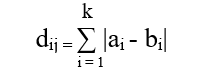# Python | Calculate City Block Distance

• Last Updated : 11 May, 2020

City block distance is generally calculated between 2-coordinates of a paired object. It is the summation of absolute difference between 2-coordinates. The city block distance of 2-points a and b with k dimension is mathematically calculated using below formula:In this article two solution are explained for this problem – one with the Python code and the other one with the use of a predefined method.

Examples:

Input:

array1 = [1, 2, 13, 5]
array2 = [1, 27, 3, 4]
Output:
The CityBlock distance between 2 array is: 36

Input:

a = [34, 44, 89, 81, 67, 49, 33, 59]
b = [98, 34, 23, 12, 233, 23, 44]
Output:
The CityBlock distance between 2 array is: 412

Code: Python scratch code to calculate the distance using the above formula.

 `import` `numpy as np`` ` `def` `cityblock_distance(A, B):`` ` `    ``result ``=` `np.``sum``([``abs``(a ``-` `b) ``for` `(a, b) ``in` `zip``(A, B)])``    ``return` `result`` ` `if` `__name__``=``=` `"__main__"``:`` ` `    ``array1 ``=` `[``1``, ``2``, ``13``, ``5``]``    ``array2 ``=` `[``1``, ``27``, ``3``, ``4``]`` ` `    ``result ``=` `cityblock_distance(array1, array2)`` ` `    ``print``(``"The CityBlock distance between 2 arrays is:"``, result)`

Output:

```The CityBlock distance between 2 arrays is: 36
```

There is no restriction for the same size of arrays. So, it’s easy to calculate City Block distance between 2-coordinates using above formula but we can calculate the same using predefined method for arrays having 2-dimension or more.

Code: Python code using the predefined method to calculate the distance using the above formula.

 `# Import necessary package``import` `scipy.spatial.distance as d`` ` `def` `CityBlock_distance(A, B):`` ` `    ``result ``=` `d.cdist(A, B, ``'cityblock'``)``    ``return` `result`` ` `if` `__name__``=``=` `"__main__"``:`` ` `    ``mat1 ``=` `[[``1``, ``2``, ``13``, ``5``], [``2``, ``3``, ``4``, ``5``]]``    ``mat2 ``=` `[[``1``, ``27``, ``3``, ``4``], [``8``, ``6``, ``9``, ``3``]]`` ` `    ``result ``=` `CityBlock_distance(mat1, mat2)`` ` `    ``print``(result)`

Output:

```[[ 36.  17.]
[ 27.  16.]]
```

My Personal Notes arrow_drop_up